# 高等数学(上册)第一章：函数与映射

• 授课教师：史悦
• 教材：

• 公邮：[email protected] 密码：2015101

# 函数与极限

## 实数的重要性质

### 有序性

• 对于任意的两个实数$a,b$,$a>b,a<b,a=b$，三者比必居其一且只是其一
• 若$a\leq b,a\geq b$,则$a=b$.
• a,b,c为实数

### 稠密性

• 任意性

• 推论

对于任意两实数$a<b$，

都存在实数$\delta >0$，

使$a<b<\delta$。

• 连续性

## 集合与平面集

### 领域

$a与\delta(\delta>0)$是两个实数，数集${x||x-a|<\delta}$称为点$a$的领域。点$a$称为领域中心，$\delta$称为领域半径。

## 常用不等式

• 绝对值不等式

• 三角不等式

• 平均值不等式

则有：

• 柯西——施瓦兹不等式

设$a_i,b_i$是实数$(i=1,2,3,4,\cdots,n)$

## 函数的概念

• 单值函数
• 多值函数

### 定义二

• 分段函数

• 取整函数$y=[x]$,表示不超过$x$的最大整数。

• 迪利克雷函数

• 取最值函数

## 函数的初等函数

• 函数的有界性

$f(x)$在$i$上有界$\iff$$f(x)在i$上既有上界又有下界。

• 无界性

若$I\in D,\forall m>0,\exists x_0 \in I$,使$|f(x_0)|>m$，则$f(x)$在$I$上无界。

• 单调性

• 奇偶性

• 周期性

• 和差

## 初等函数

• 幂函数：$y=x^\mu(\mu 时常数)$
• 指数函数：$y=a^x(a>0,且a\neq 1)$
• 对数函数：$y=\log_ax,(a>0,a\neq1)$
• 三角函数：$y=\sin x,y=\cos x,y=\tan x$
• 反三角函数：$y=\arcsin x,y=\arccos x,y=\arctan x$

# 函数的极限## 函数极限的性质# 无穷大与无穷小

### 定义一# 极限运算法则

## 定理

• 两个无穷小的和时无穷小
• 有界函数与无穷小的乘积时无穷小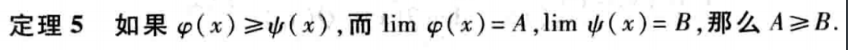# 极限存在准则与俩个重要极限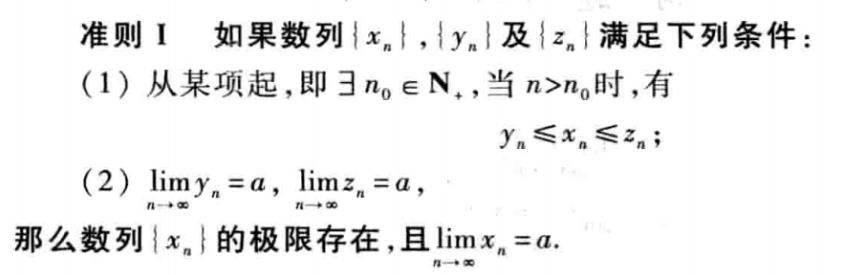### 准则二# 无穷小的比较# 函数的间断性与间断点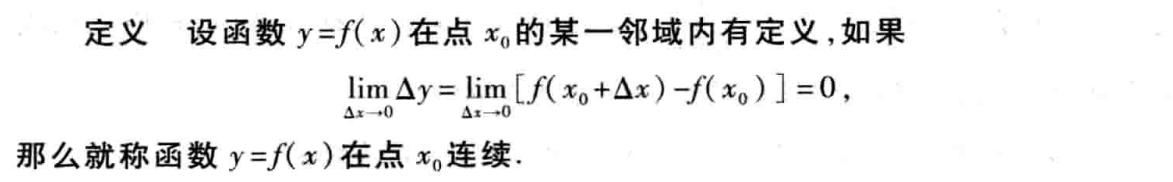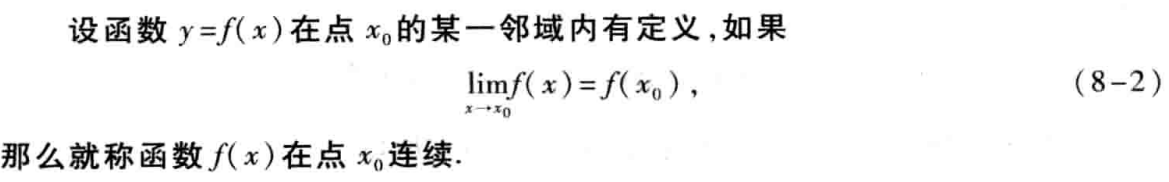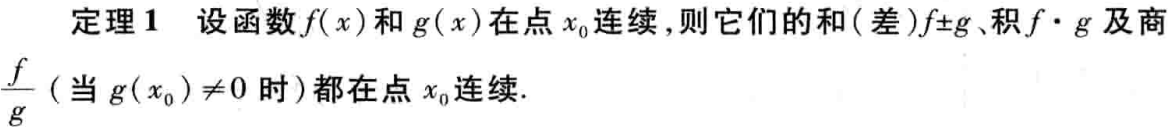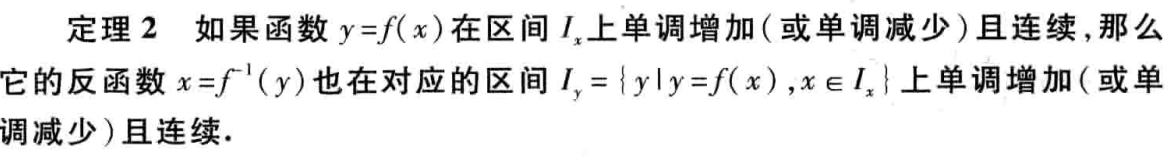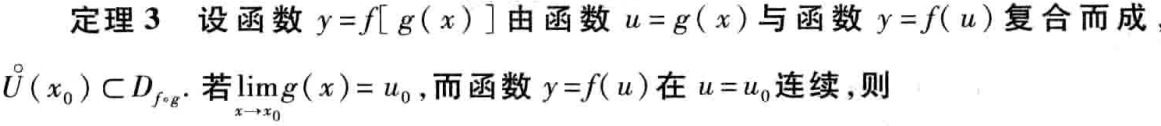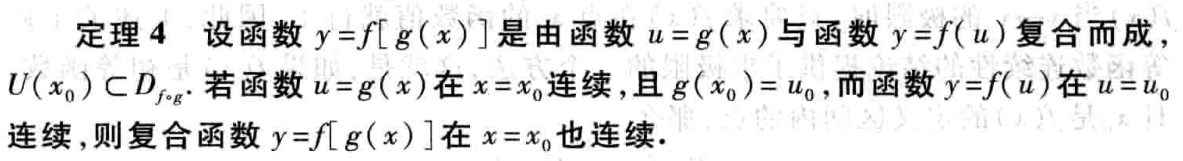# 闭区间上连续函数的性质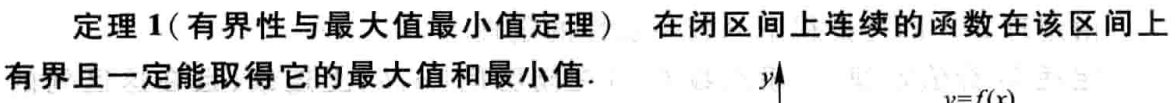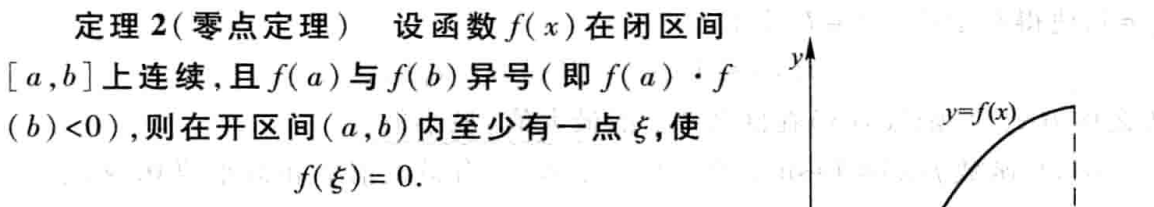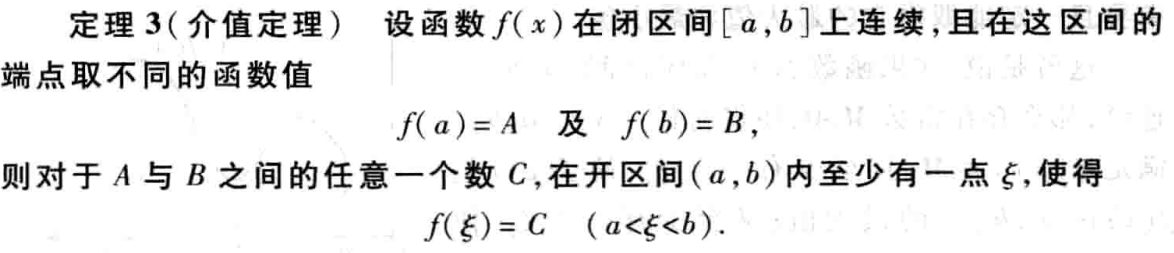## 一致连续性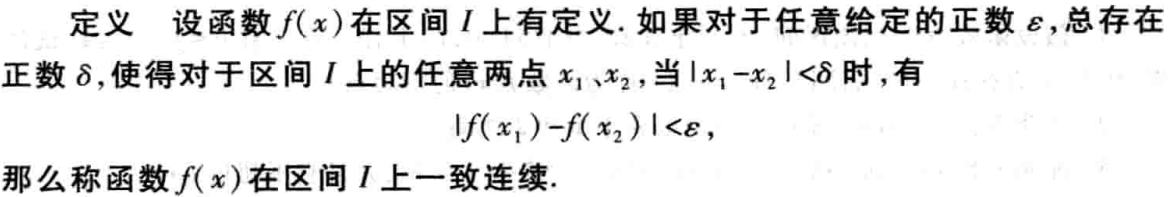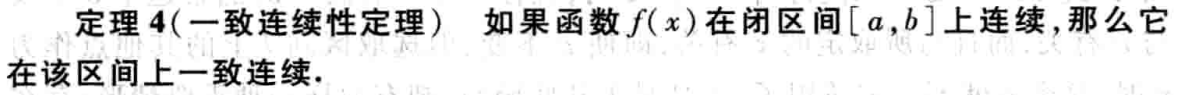## Final

If this post is helpful to you, why not reward me?
Author:
Reprint policy: All articles in this blog are used except for special statements CC BY 4.0 reprint polocy. If reproduced, please indicate source Gatsby !
Previousrequirments.txt的用法

2020-01-09
NextDcker系列(2):dcker命令

2020-01-03
TOC